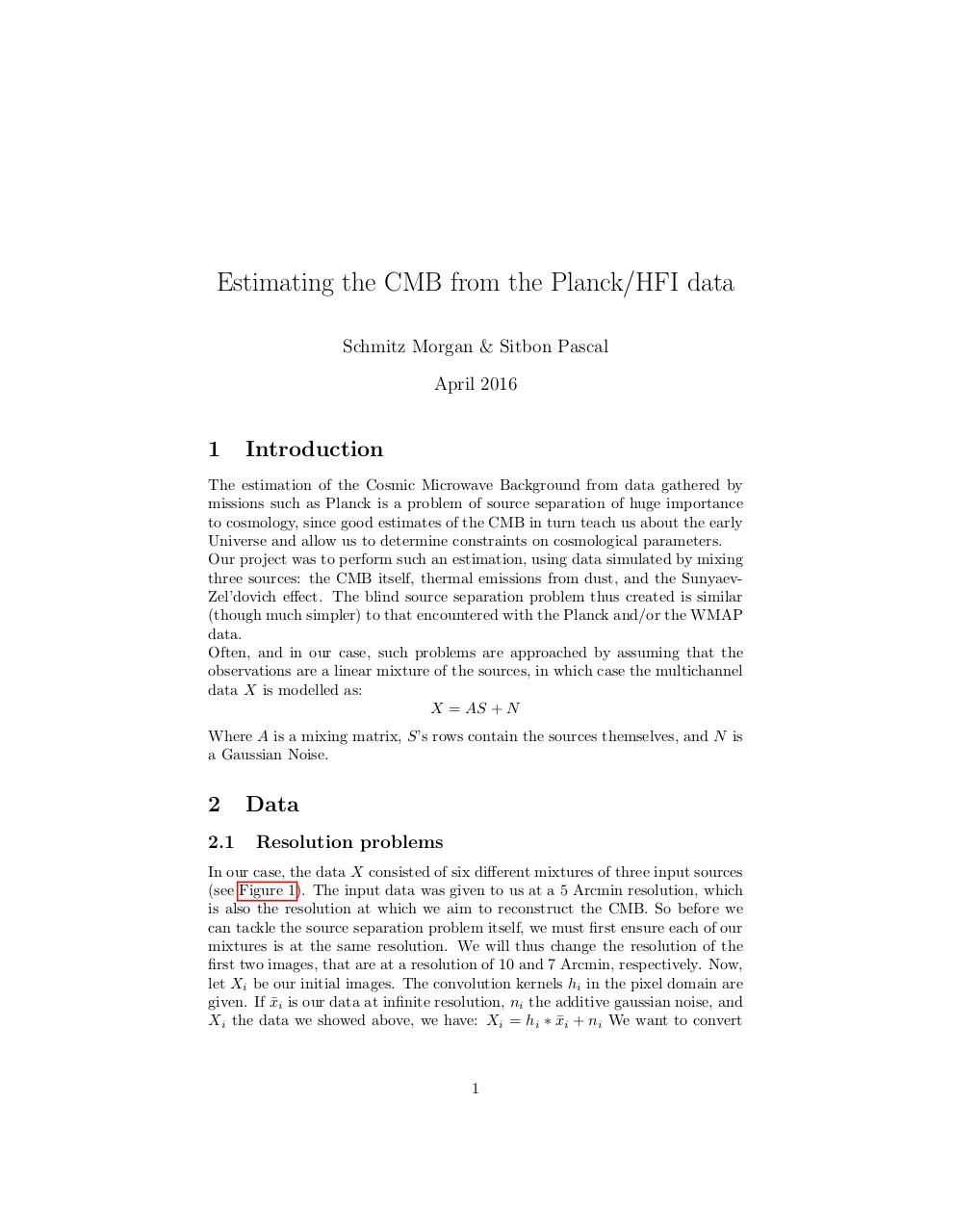# Report SchmitzSitbon.pdfPage 1 2 3 4 5 6 7 8 9 10

#### Text preview

Estimating the CMB from the Planck/HFI data
Schmitz Morgan &amp; Sitbon Pascal
April 2016

1

Introduction

The estimation of the Cosmic Microwave Background from data gathered by
missions such as Planck is a problem of source separation of huge importance
to cosmology, since good estimates of the CMB in turn teach us about the early
Universe and allow us to determine constraints on cosmological parameters.
Our project was to perform such an estimation, using data simulated by mixing
three sources: the CMB itself, thermal emissions from dust, and the SunyaevZel’dovich effect. The blind source separation problem thus created is similar
(though much simpler) to that encountered with the Planck and/or the WMAP
data.
Often, and in our case, such problems are approached by assuming that the
observations are a linear mixture of the sources, in which case the multichannel
data X is modelled as:
X = AS + N
Where A is a mixing matrix, S’s rows contain the sources themselves, and N is
a Gaussian Noise.

2
2.1

Data
Resolution problems

In our case, the data X consisted of six different mixtures of three input sources
(see Figure 1). The input data was given to us at a 5 Arcmin resolution, which
is also the resolution at which we aim to reconstruct the CMB. So before we
can tackle the source separation problem itself, we must first ensure each of our
mixtures is at the same resolution. We will thus change the resolution of the
first two images, that are at a resolution of 10 and 7 Arcmin, respectively. Now,
let Xi be our initial images. The convolution kernels hi in the pixel domain are
given. If x
¯i is our data at infinite resolution, ni the additive gaussian noise, and
Xi the data we showed above, we have: Xi = hi ∗ x
¯i + ni We want to convert

1